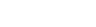# Simplifying Math with Substitution on the GMAT

This post discusses a strategy for simplifying complex mathematical expression on the GMAT Quantitative section.  Consider the following very challenging Data Sufficiency question.

1. What is the value of x?Take a moment to wrestle with this before reading this article, in which we will explain how to approach this.

## Complex Equations on the GMAT can be simplified!

How simple or complex an algebraic expression looks make no difference to the arithmetic operating on it.   When a mathematician looks at the following four expressions …… the mathematician sees, despite the apparent diversity, an underlying similarity: all four of these are in the form “thing cubed minus five.”  Because all four have the same form, that means if we can figure out something important about the first, we could probably use that to figure out something important about the others.  The first two expressions here are at the outer limit of what the GMAT will give you, and the latter two are well beyond GMAT math, but I list them here simply to demonstrate important skill of seeing essential mathematical patterns despite differences in complexity level.

## The question: preliminary observations

With the DS question above, it’s reasonably easy to see — both statements are going to allow multiple possible values for x.  The second statement, with a little factoring, becomes (x + 2)*(x – 1) = 0, so the solutions are x = {+1, –2}.  Two possible values, so Statement #2 by itself is not sufficient.  Even without tackling the first one, it looks like this will also have more than one possible solution, which means, by itself, it wouldn’t be sufficient either.  Right away, without doing much at all in the way of calculations, we can eliminate choices (A) & (B) & (D), leaving only (C) & (E).  Even if we could do nothing else, we would have excellent odds at this point if we simply guessed from the remaining two answers.  Let’s talk, though, about tackling Statement #1.

## The benighted approach

Someone who is fairly comfortable with individual math rules, but who is not accustomed to thinking like a mathematician, is likely to multiply everything out in Statement #1.  First of all, there’s the danger of making in mistake in squaring the expression in the first term, but let’s assume our foot soldier gets that part right.  Then we would have ….Well, we could simplify that further, but notice: this orgy of algebra already has passed a few spots at which algebraic mistakes would be likely to happen.  Even now, we have quartic (i.e. an equation with a fourth power), which is a bit more difficult than anything the GMAT will ask of you.

## The u-substitution method

Whenever the same algebraic expression appears in two or more terms, we can always use the letter u as a kind of mathematical abbreviation.  To use the mathematical term, we are substituting u for the repeated algebraic expression.  Here, to simply the first statement, I am going to use the u-substitution …… because that is the expression repeated in two terms of statement #1.  With this substitution, statement #1 becomes ….Now, we have found the possible values of u — we have “solved for u.”  The prompt, though, is asking for the value of x.  Given these possible values of u, what are the possible values of x?Thus, statement #1 allows for four different values of x: {+1, –1, +2, –2}.  As seen above, statement #2 allows for two different values of x: {+1, –2}.  Combined, there are still possible values of x: {+1, –2}.  Thus, even with both statements combined, we are not able to determine a unique value of x and give a definitive answer to the prompt question.  Even combined, the statements are insufficient.   Answer = E.

## Summary

Think like a mathematician.  Don’t simply plod through step-by-step —- step back and look at the patterns, and use u-substitutions to make the patterns clear.  This is an absolutely invaluable approach in +700-level GMAT Quantitative problems.   Here’s a further question for practice

Also, I’ve written up some more GMAT Data Sufficiency tips.